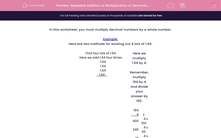# Repeated Addition or Multiplication of Decimals by a Whole Number

In this worksheet, students multiply decimals by whole numbers.Key stage:  KS 2

Curriculum topic:   Maths and Numerical Reasoning

Curriculum subtopic:   Decimals

Difficulty level:#### Worksheet Overview

In this worksheet, you must multiply decimal numbers by a whole number.

Example:

Here are two methods for working out 4 lots of 1.64.

Find four lots of 1.64.
Here we add 1.64 four times.
1.64
1.64
1.64
1.64

Here we multiply 1.64 by 4.

Remember, multiply 164 by 4

 164 4 x 400 4 x 100 240 4 x 60 16 4 x 4 656

Answer: 656 ÷ 100 = 6.56.

Your two answers should be the same, but it is quicker to multiply when using larger numbers.

### What is EdPlace?

We're your National Curriculum aligned online education content provider helping each child succeed in English, maths and science from year 1 to GCSE. With an EdPlace account you’ll be able to track and measure progress, helping each child achieve their best. We build confidence and attainment by personalising each child’s learning at a level that suits them.

Get started